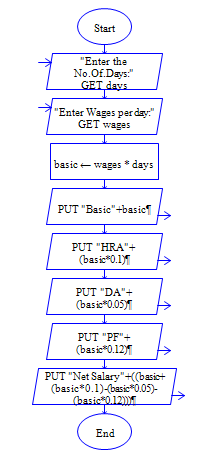# C Program to calculate Salary of Employee

Get employee wages and number of days worked from user and find Basic Pay, DA, HRA, PF and Net Pay. (Note HRA, DA and PF are 10%,5%and 12% of basicpay respectively.)

Sample Input 1:

300 30

Sample Output 1:

Basic Pay:3000 DA: 150 HRA:300 PF:360 Net Pay: 3090

#### Flow Chart DesignStrongly recommended to Solve it on your own, Don't directly go to the solution given below.

#include<stdio.h> int main() { //write your code here }

#### Program or Solution

``` #include<stdio.h> int main() { int wages; double days,basic,HRA,DA,PF,netsalary; printf("Enter daily wages and number of days worked:"); scanf("%d %lf",&wages,&days); basic=wages*days; HRA=basic*0.1; DA=basic*0.05; PF=basic*0.12; netsalary=basic+HRA+DA-PF; printf("\nBasic:%lf \nHRA:%lf \nDA:%lf \nPF:%lf \nNet Salary:%lf",basic,HRA,DA,PF,netsalary); return 0; } ```

#### Program Explanation

Get daily wage of an employee and days worked by the employee. (using scanf statement).

Calculate Basic Pay by multiplying wages & days worked

Calculate HRA by multplying basic pay and 0.1

Calculate DA by multplying basic pay and 0.05

Calculate PF by multiplying basic pay and 0.12

Calculate Net Pay by adding HRA and DA, subracting PF with Basic Pay.

Print Basic Pay, DA, HRA, PF and Net Pay (using printf statement)